## December 7, 2017

### Applying the GoldSim WGEN Model to Generate Stochastic Weather Data

Now you can let GoldSim do all the heavy lifting for you when simulating weather for your next project. A new GoldSim model example in our library lets you quickly generate the input data required to run the WGEN model, which is a stochastic weather generator built in GoldSim that creates daily stochastic time series of precipitation, temperature, and solar radiation.

This model (called WGENprep) uses time series data to automatically generate the input file you need to run WGEN. The WGEN model requires a lot of statistical information in order to run it and it can be quite a project to develop these inputs. With WGENprep, you can simply input the time series, change the simulation start and end time to correspond to the time series and the model will automatically export all the WGEN input data to an Excel spreadsheet. All you have to do is start up the WGEN model and then copy and paste the data from Excel right into the WGEN dashboard. These models were recently put to the test using some observed historical time series data gathered from the Salt Lake City airport weather station, provided by the NOAA National Centers for Environmental Information website.

The first step in this process was to gather the data I need for the site, which I chose to be Salt Lake City, Utah since that is where I was born and will be visiting there during the holidays. Perhaps I can use the WGEN model to predict the weather during my upcoming trip! As mentioned earlier, I went to the NOAA Climate Data Online to obtain most of the data used for this study. I obtained  daily historical time series data for precipitation and temperature. WGENprep will calculate a solar radiation time series for you using temperature data so you don't need to get radiation time series but if you have it available, that would be best.  Below are the time series plots showing the data required to generate the WGEN inputs.Time History Plot of data obtained from NOAA Climate Data Online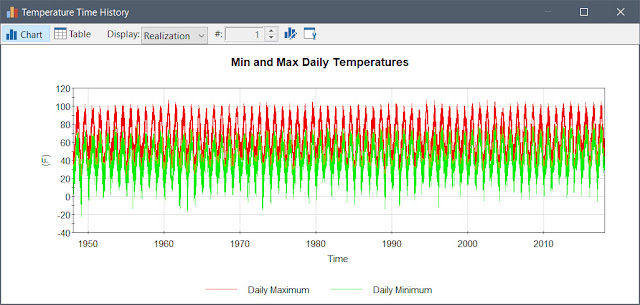Time History Plot of Min and Max Daily Temperature from NOAA Climate Data Center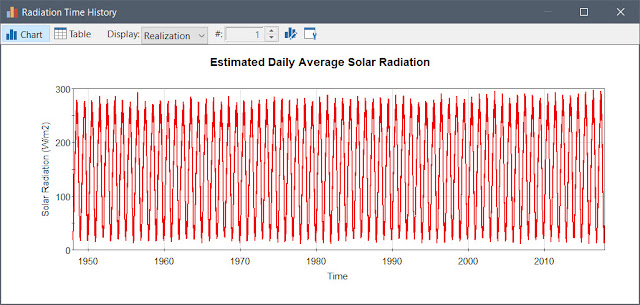Time History Plot of estimated Solar Radiation based on Temperature Data

Since my time series data started in 1948 and ends in 2017, I needed to set up my simulation settings accordingly. With this done, I ran the model, which generates totals, averages, and other statistical information from the time series inputs. The functions used to calculate the monthly totals and averages were borrowed from this monthly-annual total model example currently available for download in our library. Some of these are shown in the bar charts below. This model is capable of producing many other result charts like these.Average Monthly Results Generated by WGENprep
At the end of the simulation, the Excel file called "WGEN_Input.xlsx" is updated automatically with the inputs I used to run the WGEN model. The excel file can be obtained from this page. The Excel file looks like this:Screen capture of the Excel input file generated by WGENprep

The final step is to run the WGEN model with the data saved in the Excel file. You can obtain WGEN from our library here. In the dashboard, you will see tables of inputs with labels that match the input labels in WGEN_Inputs.xlsx and the data is in pretty much the same order so it is very easy to simply copy and paste the data. Once the model was populated with the new data as shown below, I simply ran the model for 100 realizations to produce probabilistic weather results.Screen Capture of WGEN with Input Obtained from WGENprep
This model allows you to generate probabilistic time histories of stochastically driven precipitation, temperature, and solar radiation, which are all useful for water balance models. Some of the probabilistic outputs are shown below.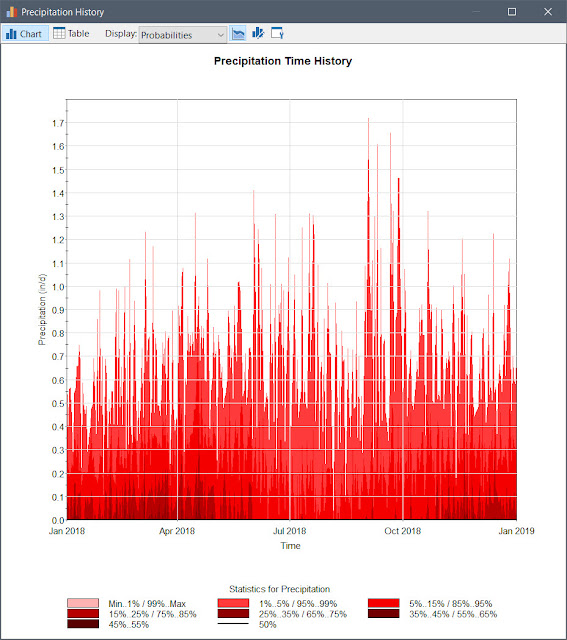Probabilistic Time History of Precipitation generated by WGEN in GoldSim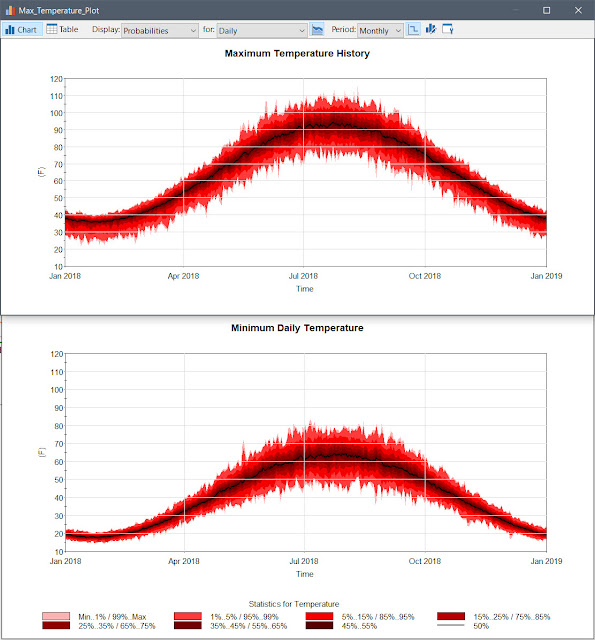Probabilistic Time History of Min and Max Daily Temperatures generated by WGEN in GoldSim

The mean time history results were compared against the mean daily observed time histories for verification, which as you can see in the charts below, matches quite well.Validation Plot comparing mean daily temperature from historical data and stochastic simulation resultsValidation Plot comparing mean daily precipitation from historical data and stochastic simulation results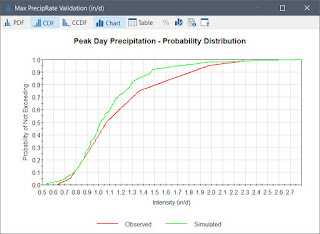Validation Plot comparing Peak Day Precipitation - Probability Distribution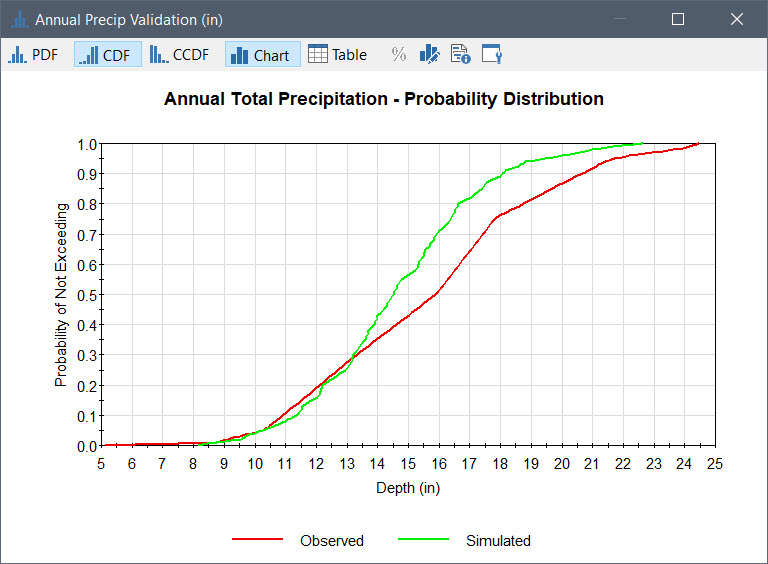Validation Plot comparing Annual Total Precipitation - Probability DistributionValidation Plot comparing Annual Wet Days - Probability Distribution
I wasn't satisfied with the calibration that resulted in the 3 plots shown above so I decided to try incorporating some auto-correlation to the 2 precipitation stochastic elements found in WGEN. It should be noted that auto-correlation of stochastic inputs is not documented so this should be considered experimental at this point. Below are the same charts but with the following stochstic inputs having auto-correlation of  0.6 and 0.4 respectively: Precip_every_day and Wet_if_less_than_prob.Validation Plot comparing Peak Day Precipitation - Probability DistributionValidation Plot comparing Annual Total Precipitation - Probability Distribution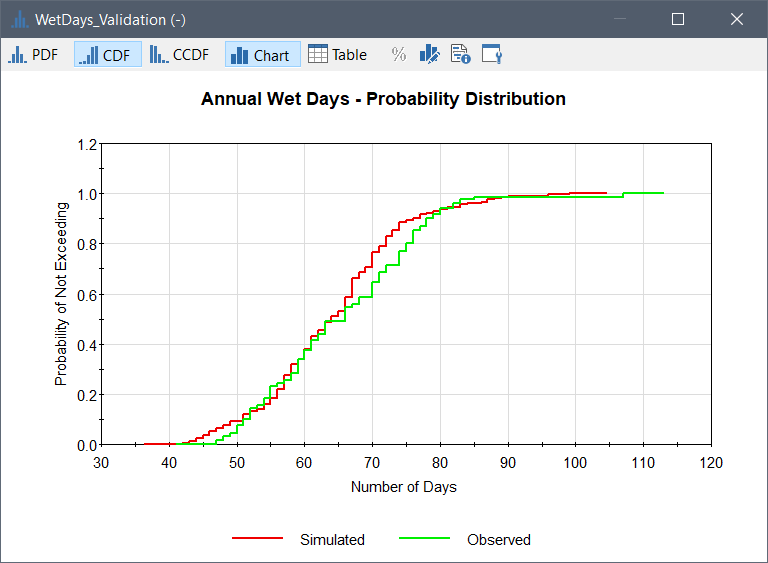Validation Plot comparing Annual Wet Days - Probability Distribution

Now you can generate stochastic weather data with just the historic time series information using this process. In fact, you could link the models together to bypass the need for Excel to better streamline the process.

The WGEN model is a very effective way to easily simulate synthetic rainfall and temperature time series as seen in the plot below.

I hope this was useful for you. If you have any questions about the process or the model files, please feel free to let us know!

1.Hi, if you were to decide that the rain probability plots shown at the end indicated a poor calibration, what would you do to improve the model?

1.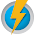Good question. If you look at the functions behind the rainfall generator of WGEN, you will see it uses a Gamma distribution with a first-order Markov chain to vary the rain intensity day by day. For WGEN, a distribution with a minimum number of parameters was needed to minimize the problem of defining the parameters for a large number of locations. I think the first step I would make at improving the calibration would be to further analyze the observed rain variability to see how closely it fits to a gamma distribution. Perhaps you could try changing the distribution. I hope this helps!

2.I just tried adding some autocorrelation to the precipitation rate and wet/dry day switch stochastics and found a much better correlation. Please see the revision above.